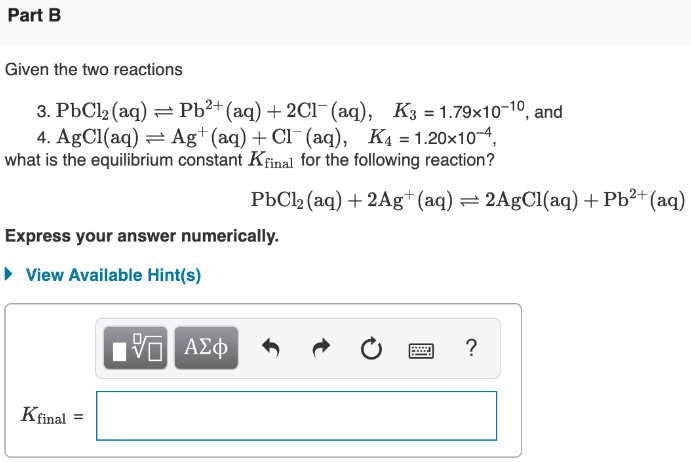# Given the two reactions: 3. PbCl2(aq) ⇌ Pb^2+(aq) + 2Cl^-(aq), K3 = 1.79x10^-10, and 4. AgCl(aq) ⇌ Ag^+(aq) + Cl^-(aq), K4 = 1.20x10^4 what is the equilibrium constant Kfinal for the following reaction? PbCl2(aq) + 2Ag^+(aq) ⇌ 2AgCl(aq) + Pb^2+(aq) Express your answer numerically.